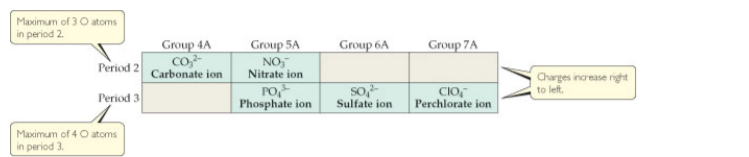# Problem: Common oxyanions. The composition and charges of common oxyanions are related to their location in the periodic table. (a) Predict the formula for the borate ion, assuming it contains a single B atom and follows the trends shown in the figure.

###### FREE Expert Solution

We are asked to predict the formula for the borate ion, assuming it contains a single B atom and follows the trends shown in the figure.

We can see in the trend that elements of the same groups will have the same number of oxygen atoms.

83% (183 ratings)###### Problem Details

Common oxyanions. The composition and charges of common oxyanions are related to their location in the periodic table.(a) Predict the formula for the borate ion, assuming it contains a single B atom and follows the trends shown in the figure.